Custom SearchMOTION IN A CIRCLE Consider the parametric equations x = r cos t and y = r sin t These equations describe the position of a point (x,y) at any time, t. They can be transposed into a single equation by squaring both sides of each equation to obtain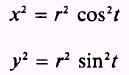and adding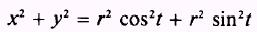Rearranging, we have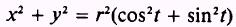butso that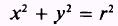which is. the equation of a circle. This means that if various values were assigned to t and the corresponding values of x and y were calculated and plotted, the result would be a circle. In other words, the point (x,y) moves in a circular path. Using this example again, that is and x = r cos t y = r sin t and given that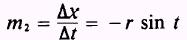and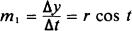we are able to express the slope at any point on the circle in terms of t. NOTE: We may find the expressions forand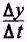by using calculus, but we will accept them for the present without proof. If we know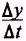and, we may find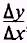which is the slope of a curve at any point. That is,By substituting, we findIn terms of a parameter, we see that m = -cot t while in terms of rectangular coordinates, we know from trigonometry thatIntegrated Publishing, Inc. - A (SDVOSB) Service Disabled Veteran Owned Small Business